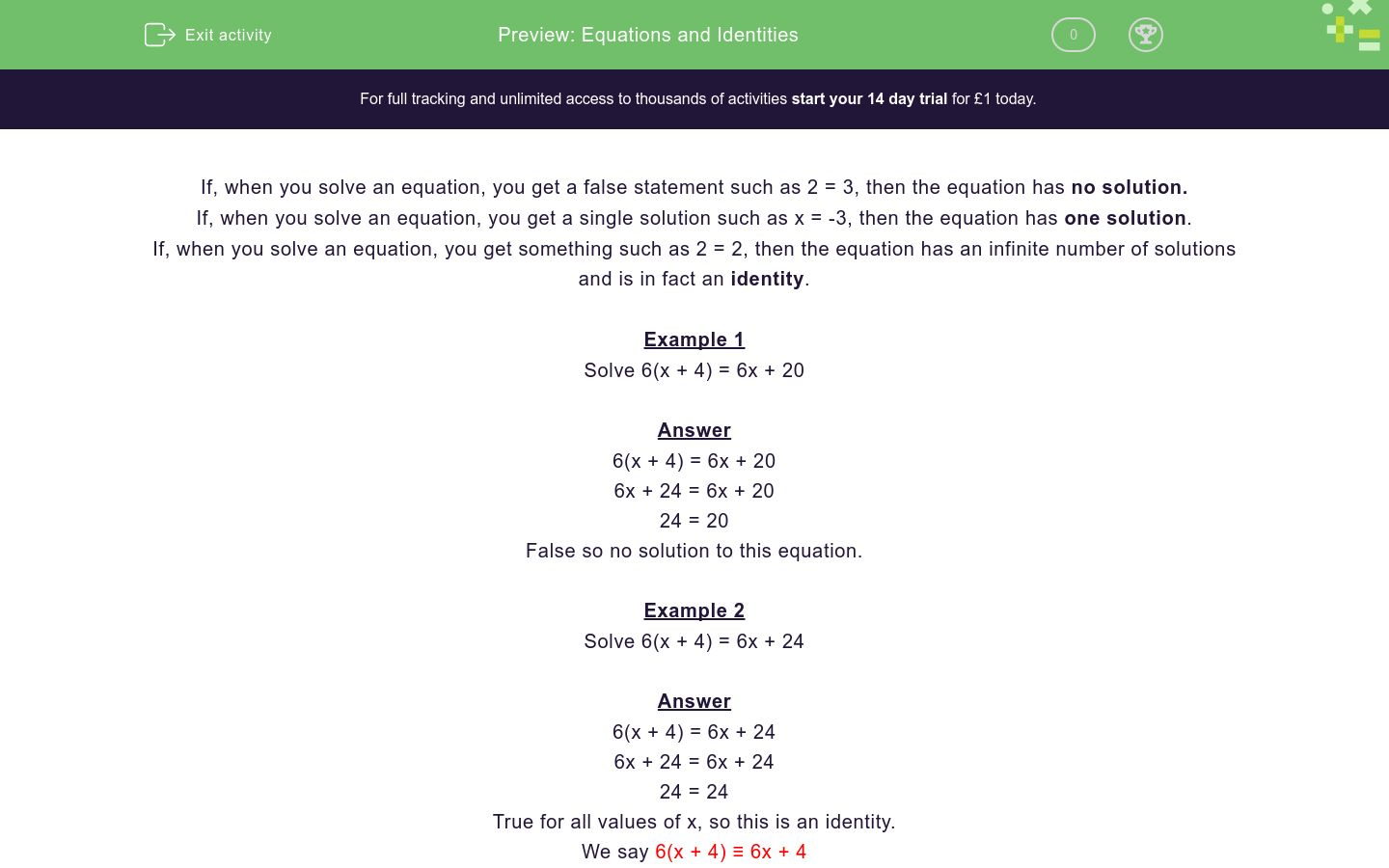# Equations and Identities

In this worksheet, students identify identities and equations.Key stage:  KS 4

Curriculum topic:  Algebra

Curriculum subtopic:  Know the Difference Between an Equation and an Identity

Difficulty level:### QUESTION 1 of 10

If, when you solve an equation, you get a false statement such as 2 = 3, then the equation has no solution.

If, when you solve an equation, you get a single solution such as x = -3, then the equation has one solution.

If, when you solve an equation, you get something such as 2 = 2, then the equation has an infinite number of solutions and is in fact an identity.

Example 1

Solve 6(x + 4) = 6x + 20

6(x + 4) = 6x + 20

6x + 24 = 6x + 20

24 = 20

False so no solution to this equation.

Example 2

Solve 6(x + 4) = 6x + 24

6(x + 4) = 6x + 24

6x + 24 = 6x + 24

24 = 24

True for all values of x, so this is an identity.

We say 6(x + 4) ≡ 6x + 4

Example 3

Solve 6(x + 4) = 5x + 20

6(x + 4) = 5x + 20

6x + 24 = 5x + 20

x = -4

One solution to this equation.

Is this an equation with one solution, an equation with no solutions or an identity?

3x - 3x = 0

Equation with one solution

Equation with no solutions

Identity

Is this an equation with one solution, an equation with no solutions or an identity?

3x - x = 0

Equation with one solution

Equation with no solutions

Identity

Is this an equation with one solution, an equation with no solutions or an identity?

3x - x = 3

Equation with one solution

Equation with no solutions

Identity

Is this an equation with one solution, an equation with no solutions or an identity?

3x = 3x + 4

Equation with one solution

Equation with no solutions

Identity

Is this an equation with one solution, an equation with no solutions or an identity?

3x = 3(x + 4)

Equation with one solution

Equation with no solutions

Identity

Is this an equation with one solution, an equation with no solutions or an identity?

3x + 12 = 3(x + 4)

Equation with one solution

Equation with no solutions

Identity

Is this an equation with one solution, an equation with no solutions or an identity?

3x + 12 = 4(x + 4)

Equation with one solution

Equation with no solutions

Identity

Is this an equation with one solution, an equation with no solutions or an identity?

3x + 12 = 12 + 2x + x

Equation with one solution

Equation with no solutions

Identity

Is this an equation with one solution, an equation with no solutions or an identity?

-3x - 12 = 12 + 2x + x

Equation with one solution

Equation with no solutions

Identity

Is this an equation with one solution, an equation with no solutions or an identity?

-3x - 12 = 12 - 2x - x

Equation with one solution

Equation with no solutions

Identity

• Question 1

Is this an equation with one solution, an equation with no solutions or an identity?

3x - 3x = 0

Identity
• Question 2

Is this an equation with one solution, an equation with no solutions or an identity?

3x - x = 0

Equation with one solution
• Question 3

Is this an equation with one solution, an equation with no solutions or an identity?

3x - x = 3

Equation with one solution
• Question 4

Is this an equation with one solution, an equation with no solutions or an identity?

3x = 3x + 4

Equation with no solutions
• Question 5

Is this an equation with one solution, an equation with no solutions or an identity?

3x = 3(x + 4)

Equation with no solutions
• Question 6

Is this an equation with one solution, an equation with no solutions or an identity?

3x + 12 = 3(x + 4)

Identity
• Question 7

Is this an equation with one solution, an equation with no solutions or an identity?

3x + 12 = 4(x + 4)

Equation with one solution
• Question 8

Is this an equation with one solution, an equation with no solutions or an identity?

3x + 12 = 12 + 2x + x

Identity
• Question 9

Is this an equation with one solution, an equation with no solutions or an identity?

-3x - 12 = 12 + 2x + x

Equation with one solution
• Question 10

Is this an equation with one solution, an equation with no solutions or an identity?

-3x - 12 = 12 - 2x - x